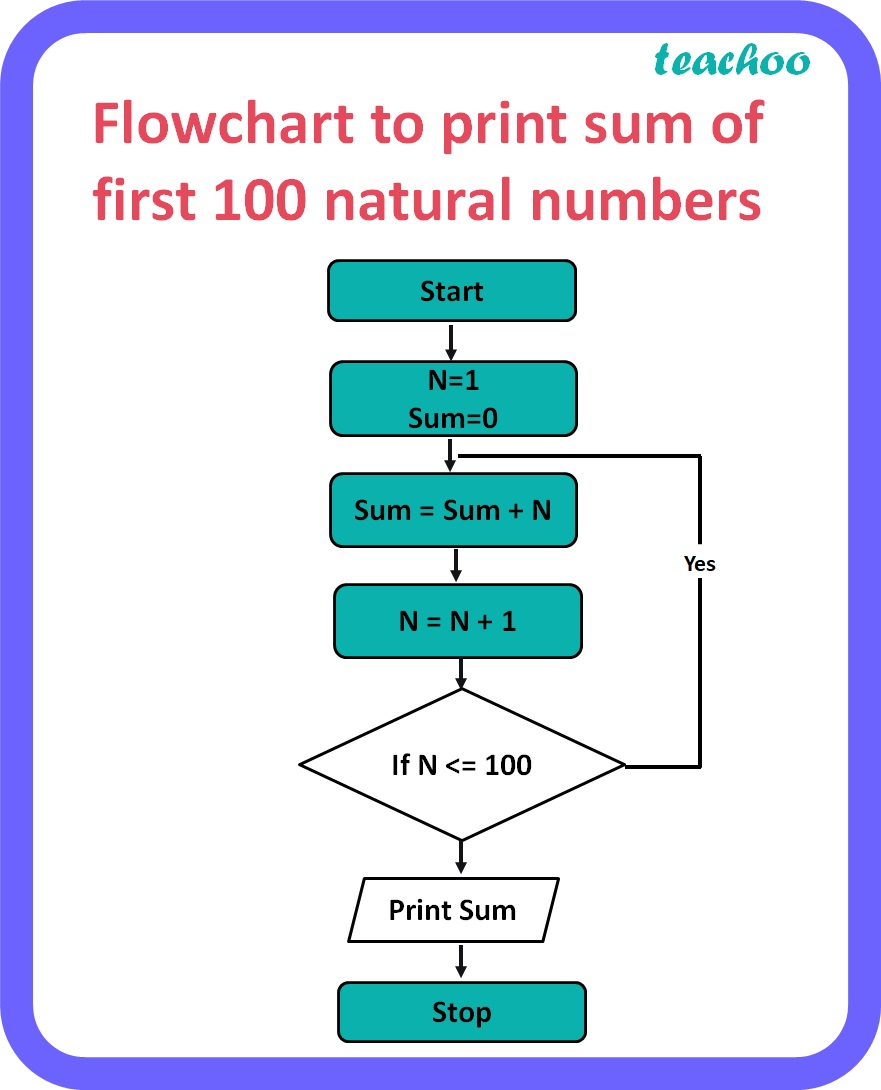Short Answer Type Questions (2 Marks each)

Computer Science - Class 11
Chapter 4 Class 11 - Introduction to Problem Solving

## Draw a flowchart to print sum of first 100 natural numbers.

Flowchart to print sum of first 100 natural numbers:Learn in your speed, with individual attention - Teachoo Maths 1-on-1 Class

### Transcript

Flowchart to print sum of first 100 natural numbers Start N=1 Sum=0 Sum = Sum + N N = N + 1 If N <= 100 Print Sum Stop